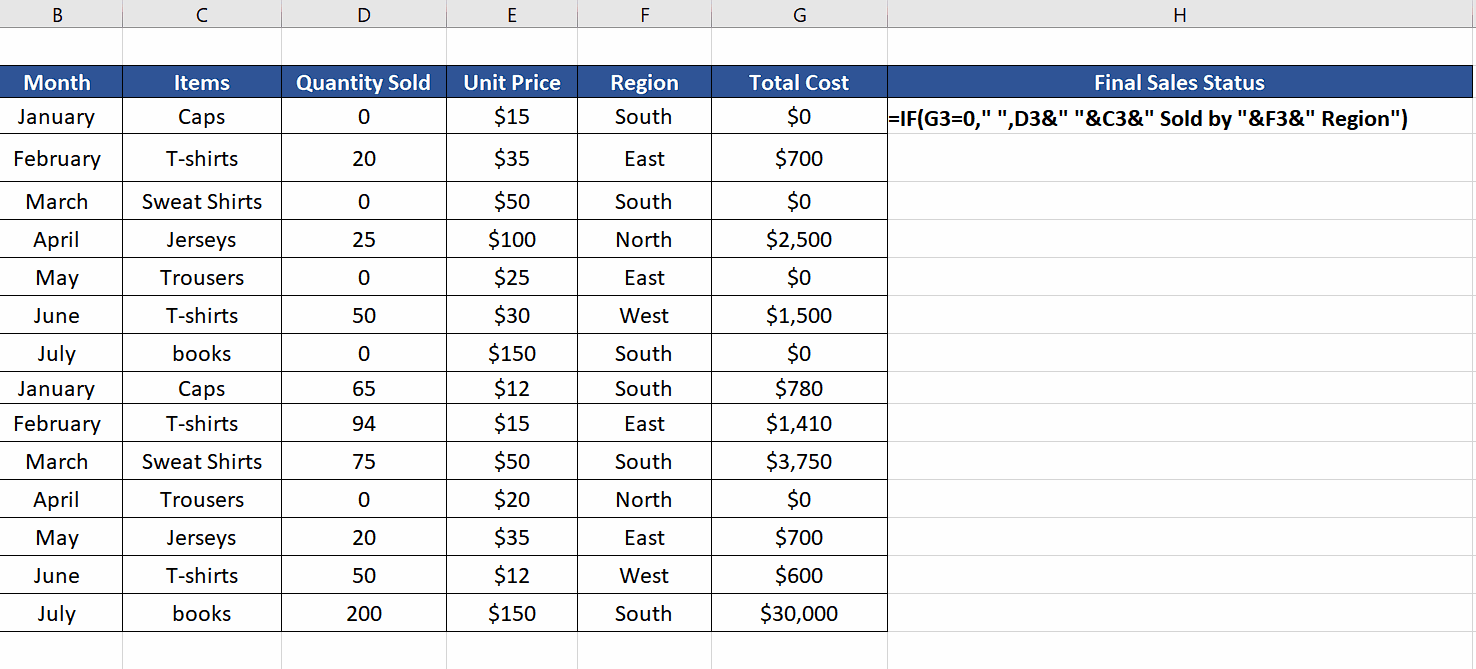# How to keep cell blank if another cell is 0 in Excel

Therefore, in this tutorial, we will learn how to implement this condition by using IF function. You may follow How to use IF-Else in Excel to understand the syntax and application of IF function.

Let’s see how to implement these methods in the steps below. Let’s look at the dataset at hand. It consists of sales data from various regions. We wish to check the sales of each item and if any sales is Zero then a blank should be displayed in the final sales status, otherwise a meaningful message should be displayed e.g., “20 T-shirt sold by East Region”. We’ll combine data from all cells in IF function using the simple & ampersand sign.Excel is a very powerful software for mathematical computation but at the same time it provides us with a lot of functions to make logical decisions based upon the values of cells. One such situation is where you want to check if a cell contains a 0 then keep the resultant cell blank. For example, if a calculation involving the cell produces a result of 0, then there may be no need to display that result in the output if it does not provide any useful information. This can help make the resulting data easier to understand and interpret. Additionally, if the result of a calculation involving a cell that is 0 is itself 0, then displaying a blank result cell can help avoid potential errors or misunderstandings in the data.

### Step 1 – Create a formula using IF function– We’ll use the following formula
=IF(G3=0,” “,D3&” “&C3&” Sold by “&F3&” Region”)

– Press Enter to implement the formula and get the first result. We’ll get a blank because the first sales is Zero.

### Step 2 – Implement the formula to the whole column– Now move the mouse over to the bottom right corner of the first cell, where we implemented the formula.

– When the cursor changes the shape to a black plus sign, double click with the mouse.

– This will copy the formula to the whole column and we’ll get our desired results as shown above.